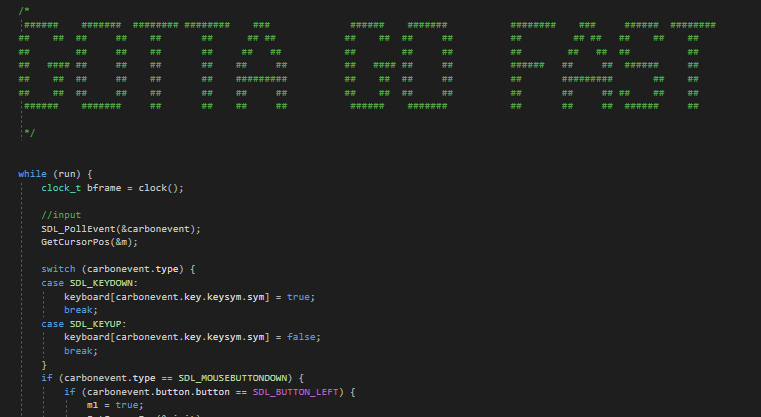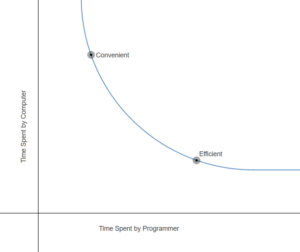Sunday, June 26, 2022

The Roundup is the Jesuit Dallas Student Voice and Newspaper since 1942. Learn about us.

More
Home Academics Computer Science When Code is Too Slow

### When Code is Too SlowOne of the challenges inherent to computer science is balancing the work done by the programmer in writing the code and the work done by the hardware in running the code. For instance, a programmer can write software to find the tenth decimal of pi in a few minutes. However, he could spend more time making the code easier for the computer to run.

Thus, there is an inverse relationship between the time spent programming and the time the computer works through the code. I think it looks something like this:Convenient solutions tend to be messy, difficult to change, and slow for the computer to run, whereas optimized solutions tend to be readable, easily modified, and quicker for the computer.

## An example

To demonstrate, I’ve written a program drawing shadows from a light bulb and some boxes. My initial idea was to create a black texture and draw tons of white lines from the light bulb erasing the shadow. The lines would continue until hitting the edges of the screen or a box.

This was very slow for the computer; it took an average time of 56.4 milliseconds to calculate all of the shadows.

This code wasted a lot of time. Because the light lines farther from the bulb spread out further, the program had to compute more lines than necessary close to the bulb in order to have enough when far away.

## A more efficient solution

Instead of checking for every pixel individually, categorize pixels together by drawing quadrilaterals between each face of the boxes and edges of the screen. The point on the edge of the screen will be collinear with the light bulb’s position. Any pixel in a quadrilateral is shaded.

This solution is much faster, taking an average of 11.4 milliseconds to draw all of the shadows.

## Does that mean convenient solutions are useless?

Absolutely not.

If the convenient solution is not much slower than the optimized one, it may not matter to programmers. For instance, if I just wanted to draw one picture of the shadows, all of the work put into the optimized answer would only save me 45 milliseconds.

Furthermore, programmers can use the convenient solution to test the optimized solution. The optimized code might be unintuitive and difficult to interpret, but still meant to provide identical results. If it doesn’t, it’s worse than the convenient code.

Finally, notice how my first idea for the light bulb problem was physically accurate, simulating individual rays of light instead of categorizing areas as shadow or light. This could be expanded to account for incredibly detailed light bouncing, scattering, absorption, etc. For this reason, Disney uses a method more similar to my initial approach.

Part of the challenge is knowing which approach to use. When I was writing my rendering software, I decided to use inefficient solutions if they were only done once when the program was launched, and work to optimize code if it was run constantly. So, the code to load models was downright lazy, but it didn’t matter, because the program was only about a second slower to launch. However, when actually drawing the mesh, I worked hard to find efficient solutions, because that would have a constant impact on the software. Enough laziness in code constantly run would eventually make the software unusable.

If you are interested in optimization or want to see the source code, feel free to talk to me or send an email! My email is 19046@jcpstudents.org.

## An explanation for nerds

This is the crux of the efficient code:

``````//two points for end of quad shadow
point p5;
point p6;

//find slope and y intercept
float m;
float b;

m = (Boxes[i].p1.y - bulb.y) / (Boxes[i].p1.x - bulb.x);
b = Boxes[i].p1.y - (m * Boxes[i].p1.x);

//set x value to be at edge of screen in appropriate direction
p5.x = 1440;
if (bulb.x > Boxes[i].p1.x) {
p5.x = 0;
}

//calculate y value from x, b, and m
p5.y = m * p5.x + b;

//repeat for second point
m = (Boxes[i].p2.y - bulb.y) / (Boxes[i].p2.x - bulb.x);
b = Boxes[i].p2.y - (m * Boxes[i].p2.x);
p6.x = 1440;
if (bulb.x > Boxes[i].p2.x) {
p6.x = 0;
}
p6.y = m * p6.x + b;